2022-06-19
###### Evaluation metrics for Multi-Label Classification with Python codes
2022-06-19The F1 score (aka F-measure) is a popular metric for evaluating the performance of a classification model. In the case of multi-class classification, we adopt averaging methods for F1 score calculation, resulting in a set of different average scores (macro, weighted, micro) in the classification report. This post looks at the meaning of these averages, how to calculate them, and which one to choose for reporting.

## Contents

• Basics
• Setting the Motivating Example
• Macro Average
• Weighted Average
• Micro Average
• Which average should I choose?

## Basics

Any individual associated with Data Science must have heard of the terms Precision and Recall. We come across these terms quite often whenever we are stuck with any classification problem. If you have spent some time exploring Data Science, you must have an idea of how accuracy alone can be misleading many times in analyzing the performance of any model. I won’t be discussing that here.

The formulae for Precision and Recall won’t be alien to you either. Though let’s have a recap:

### Precision

Layman definition: Of all the positive predictions I made, how many of them are truly positive?

Calculation: Number of True Positives (TP) divided by the Total Number of True Positives (TP) and False Positives (FP).

### Recall

Layman definition: Of all the actual positive examples out there, how many of them did I correctly predict to be positive?

Calculation: Number of True Positives (TP) divided by the Total Number of True Positives (TP) and False Negatives (FN).

If you compare the formula for precision and recall, you will notice both look similar. The only difference is the second term of the denominator, where it is False Positive for precision but False Negative for recall.

## F1 Score

To evaluate model performance comprehensively, we should examine both precision and recall. The F1 score serves as a helpful metric that considers both of them.

Definition: Harmonic mean of precision and recall for a more balanced summarization of model performance.

Calculation:

If we express it in terms of True Positive (TP), False Positive (FP), and False Negative (FN), we get this equation: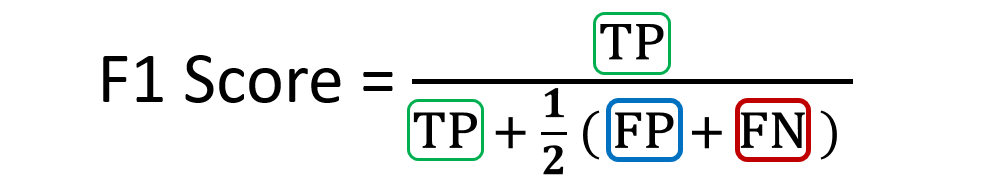The alternative equation for F1 score

These formulae can be used with only the Binary Classification problem (Something like Titanic on Kaggle where we have a ‘yes’ or ‘no’ or with problems with 2 labels for example Black or Red where we take one as 1 and the others as 0 ).

## What about Multi-Class Problems?

Like if I have a classification problem with 3 or more classes i.e Black, Red, Blue, White, etc. The above formulae won’t just fit in!!! Though calculating accuracy won’t be a problem

## Setting the Motivating Example

To illustrate the concepts of averaging F1 scores, we will use the following example in the context of this tutorial. Imagine we have trained an image classification model on a multi-class dataset containing images of three classes: Airplane, Boat, and Car.

We use this model to predict the classes of ten test set images. Here are the raw predictions: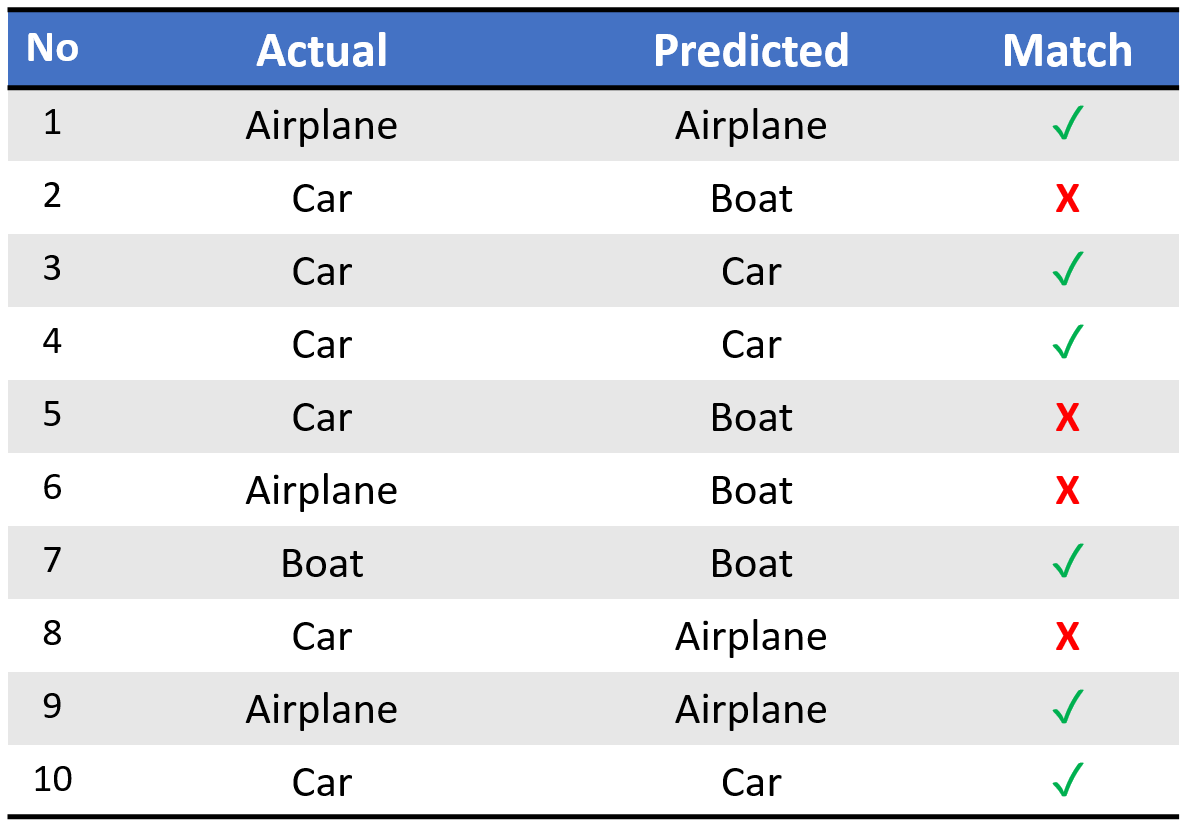Sample predictions of our demo classifier

Upon running sklearn.metrics.classification_report, we get the following classification report:

The columns (in orange) with the per-class scores (i.e. score for each class) and average scores are the focus of our discussion. We can see from the above that the dataset is imbalanced (only one out of ten test set instances is ‘Boat’). Thus the proportion of correct matches (aka accuracy) would be ineffective in assessing model performance. Instead, let us look at the confusion matrix for a holistic understanding of the model predictions.

The confusion matrix above allows us to compute the critical values of True Positive (TP), False Positive (FP), and False Negative (FN), as shown below.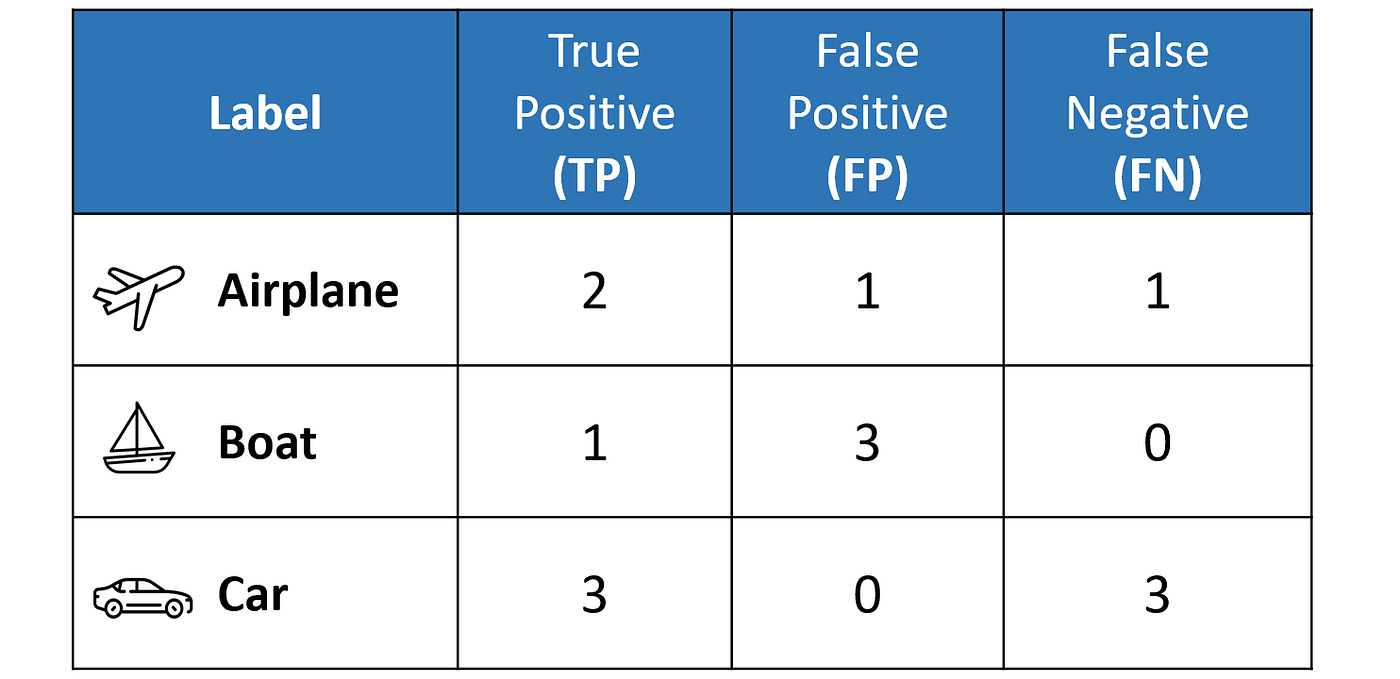Calculated TP, FP, and FN values from the confusion matrix

The above table sets us up nicely to compute the per-class values of precisionrecall, and F1 score for each of the three classes. It is important to remember that in multi-class classification, we calculate the F1 score for each class in a One-vs-Rest (OvR) approach instead of a single overall F1 score as seen in binary classification. In this OvR approach, we determine the metrics for each class separately, as if there is a different classifier for each class. Here are the per-class metrics (with the F1 score calculation displayed):

However, instead of having multiple per-class F1 scores, it would be better to average them to obtain a single number to describe overall performance.

Now, let’s discuss the averaging methods that led to the three different average F1 scores in the classification report.

## Macro Average

Macro averaging is perhaps the most straightforward among the numerous averaging methods. The macro-averaged F1 score (or macro F1 score) is computed by taking the arithmetic mean (aka unweighted mean) of all the per-class F1 scores. This method treats all classes equally regardless of their support values.

The value of 0.58 we calculated above matches the macro-averaged F1 score in our classification report.

## Weighted Average

The weighted-averaged F1 score is calculated by taking the mean of all per-class F1 scores while considering each class’s support. Support refers to the number of actual occurrences of the class in the dataset. For example, the support value of 1 in Boat means that there is only one observation with an actual label of Boat. The ‘weight’ essentially refers to the proportion of each class’s support relative to the sum of all support values.

With weighted averaging, the output average would have accounted for the contribution of each class as weighted by the number of examples of that given class. The calculated value of 0.64 tallies with the weighted-averaged F1 score in our classification report.

## Micro Average

Micro averaging computes a global average F1 score by counting the sums of the True Positives (TP), False Negatives (FN), and False Positives (FP). We first sum the respective TP, FP, and FN values across all classes and then plug them into the F1 equation to get our micro F1 score.

In the classification report, you might be wondering why our micro F1 score of 0.60 is displayed as ‘accuracy ’ and why there is NO row stating micro avg’.

The reason is that micro-averaging essentially computes the proportion of correctly classified observations out of all observations. If we think about this, this definition is in fact what we use to calculate overall accuracy. Furthermore, if we were to do micro-averaging for precision and recall, we would get the same value of 0.60.

These results mean that in multi-class classification cases where each observation has a single label, the micro-F1micro-precisionmicro-recall, and accuracy share the same value (i.e., 0.60 in this example). And this explains why the classification report only needs to display a single accuracy value, since micro-F1, micro-precision, and micro-recall also have the same value.

micro-F1 = accuracy = micro-precision = micro-recall

A more detailed explanation of this observation could be found in this post.

## Which average should I choose?

In general, if you are working with an imbalanced dataset where all classes are equally important, using the macro average would be a good choice as it treats all classes equally. It means that for our example involving the classification of airplanes, boats, and cars, we would use the macro-F1 score.

If you have an imbalanced dataset but want to assign greater contribution to classes with more examples in the dataset, then the weighted average is preferred. This is because, in weighted averaging, the contribution of each class to the F1 average is weighted by its size.

Suppose you have a balanced dataset and want an easily understandable metric for overall performance regardless of the class. In that case, you can go with accuracy, which is essentially our micro F1 score.

## Another example of calculating Precision and Recall for Multi-Class Problems

Let us first consider the situation. Assume we have a 3 Class classification problem where we need to classify emails received as Urgent, Normal, or Spam. Now let us calculate Precision and Recall for this using the below methods:

## MACRO AVERAGING

The Row labels (index) are output labels (system output) and Column labels (gold labels) depict actual labels. Hence,

• [urgent,normal]=10 means 10 normal(actual label) mails has been classified as urgent.
• [spam,urgent]=3 means 3 urgent(actual label) mails have been classified as spam

The mathematics isn’t tough here. Just a few things to consider:

• Summing over any row values gives us Precision for that class. Like precision_u=8/(8+10+1)=8/19=0.42 is the precision for class:Urgent

Similarly for precision_n(normal), precision_s(spam)

• Summing over any column gives us Recall for that class. Example:

recall_s=200/(1+50+200)=200/251=0.796. Similarly consider for recall_u (urgent) and recall_n(normal)

Now, to calculate the overall precision, average the three values obtained

## MICRO AVERAGING

Micro averaging follows the one-vs-rest approach. It calculates Precision and Recall separately for each class with True(Class predicted as Actual) and False(Classed predicted!=Actual class irrespective of which wrong class it has been predicted). The below confusion metrics for the 3 classes explain the idea better.

Now, we add all these metrics to produce the final confusion metric for the entire data i.e Pooled. Looking at cell [0,0] of Pooled matrix=Urgent[0,0] + Normal[0,0] + Spam[0,0]=8 + 60 + 200= 268

Now, using the old formula, calculating precision= TruePositive(268)/(TruePositive(268) + FalsePositive(99))=0.73

Similarly, we can calculate Recall as well.

As we can see in the above calculations the Micro average is moved by the majority class (In our case, Spam), and therefore it might not depict the performance of the model in all classes (especially minority classes like ‘Urgent’ which have fewer samples in test data). If you observe, the model performs poorly for ‘Urgent’ but the overall number obtained by micro averaging can be misleading which gives 70% precision. Though, for class urgent, the actual precision is just 42%. Hence macro averaging does have an edge over micro averaging.

## Summary

When you have a multiclass setting, the average parameter in the f1_score function needs to be one of these:

• ‘weighted’
• ‘micro’
• ‘macro’

The first one, ‘weighted’ calculates de F1 score for each class independently but when it adds them together uses a weight that depends on the number of true labels of each class: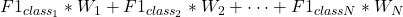therefore favoring the majority class.

‘micro’ uses the global number of TP, FN, and FP and calculates the F1 directly: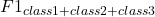no favoring any class in particular.

Finally, ‘macro’ calculates the F1 separated by class but not using weights for the aggregation: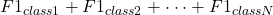which results in a bigger penalization when your model does not perform well with the minority classes.

So:

• average=micro says the function to compute f1 by considering total true positives, false negatives, and false positives (no matter the prediction for each label in the dataset)
• average=macro says the function to compute f1 for each label, and returns the average without considering the proportion for each label in the dataset.
• average=weighted says the function to compute f1 for each label, and returns the average considering the proportion for each label in the dataset.

The one to use depends on what you want to achieve. If you are worried about class imbalance I would suggest using ‘macro’. However, it might be also worthwhile implementing some of the techniques available to tackle imbalance problems such as downsampling the majority class, upsampling the minority, SMOTE, etc.

Resources:

https://towardsdatascience.com/micro-macro-weighted-averages-of-f1-score-clearly-explained-b603420b292f

https://medium.com/data-science-in-your-pocket/calculating-precision-recall-for-multi-class-classification-9055931ee229